## The Lorentz Transformation

Einstein postulated that the speed of light is the same in any inertial frame of reference. It is not possible to meet this condition if the transformation from one inertial reference frame to another is done with a universal time, that is,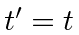. Let us study a transformation from one inertial reference frame to another that is moving with a constant velocity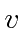in thedirection. Such a transformation is usually referred to as a boost.

Newton's motion with constant velocitytransforming to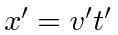requires that the transformation be linear, like a rotation. We therefore try a linear transformation in which both the position and the time transform. Since this linear transformation will mixand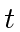, it is reasonable to try to transform quantities that have the same units 2, so we will try a dimensionless transformation ofand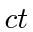.

We will work in just two dimensions,and, like a rotation in the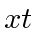plane. (For the boost along thedirection,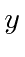andare not changed.)Assume the origin of a second inertial frame (primed), is moving at velocityin thedirection, and corresponds to the (unprimed) origin at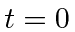. All of these choices can be made without loss of generality. The most general linear transformation ofandcan be written with four dimensionless coefficients. We will call these coefficients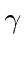,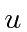,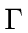, and.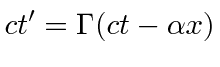By the very definition of the transformation we have that the origin of the primed frame is atin the unprimed frame. (This makes the boost clearly different from a rotation.)the given transformationdefinition of the boostSo we can identify the constantto be the velocity of the transformation.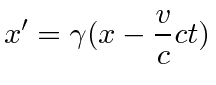The inverse of the transformation must be the same as a transformation withas the velocity of moving frame.andare scale factors that depend on the velocity of the transformation. Since there is no difference between theand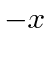directions in physics, we must use the sameandin the inverse transformation which has the same magnitude of velocity.the transformation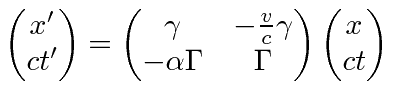transformation as matrix eq.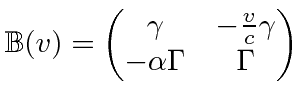transformation matrix as function ofinverse is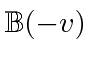exceptdepends onrhs is just inverse of 2X2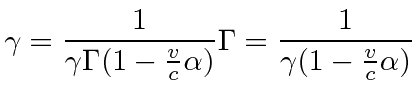upper leftlower right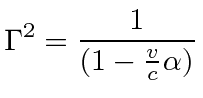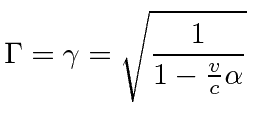must be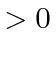plugupper left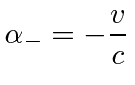lower left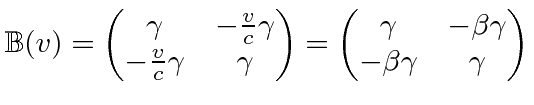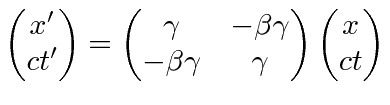the transformation now

Now, consider a pulse of light moving in thedirection emitted atandin one inertial frame. Since the origins of the two systems coincide at, this light is emitted in the primed frame with, and. At a later time, the position of the light pulse will be at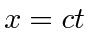. By Einstein's postulate that the speed of light is independent of inertial frame, (and by the Michelson-Morley measurement). The pulse of light should be atin the primed frame. Our transformation must give this result, so lets try it. Transforming the later position of the light pulse, we get.plug in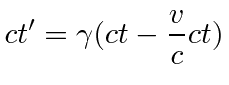plug infrom the 2 eq.

The condition that the speed of light is the same in the two reference frames is met.

We have shown that the most general transformation to a frame moving with a velocity, that is consistent with Newton's laws and the isotropy of space, and that satisfies the condition that the inverse of the transformation is a transformation with velocity, is given by: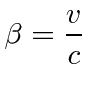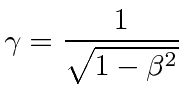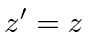This transformation also gives the result that the speed of light is independent of which inertial frame of reference we use.

It took surprisingly little physics input to derive the Lorentz transformation for the space-time coordinates.

Jim Branson 2012-10-21